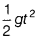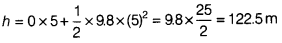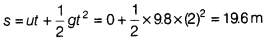Calculate the height of the bridge from the water level and the distance covered by the stone in 2 s

A stone is dropped freely in a river from a bridge. It taken 5s to touch the water surface in the river. Calculate

1. the height of the bridge from the water level
2. the distance covered by the stone in 2 s. (Take, g = 9.8 m/s2)

Given, u = 0, a = g = 9.8 m/s2, t = 5s

1. From equation of motion, h = ut +2. Distance covered by the stone in t = 2 s ⇒